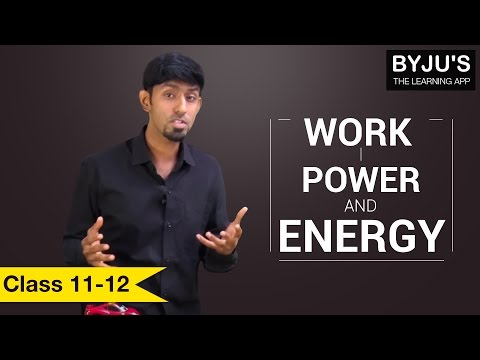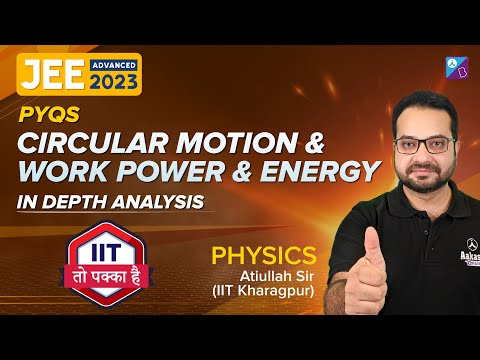# Power

Power is always dependent on work done, so if a person does work at different rates his power also differs at different times. This is where the concept of average-power comes into the picture. In this session, let us learn about power, average power, unit and formula of power.

## What is Power?

We can define power as the rate of doing work, it is the work done in unit time. The SI unit of power is Watt (W) which is joules per second (J/s). Sometimes the power of motor vehicles and other machines is given in terms of Horsepower (hp), which is approximately equal to 745.7 watts.

### What is Average Power?

We can define average power as the total energy consumed divided by the total time taken. In simple language, we can say that average power is the average amount of work done or energy converted per unit of time.

### Power Formula

Power is defined as the rate at which work is done upon an object. Power is a time-based quantity. Which is related to how fast a job is done. The formula for power is mentioned below.

Power = Work / time

P = W / t

### Unit of Power

The unit for standard metric work is the Joule and the standard metric unit for time is the second, so the standard metric unit for power is a Joule / second, defined as a Watt and abbreviated W.
See the video below to understand electric power and energy### Solved Example

Q1. A 60-watt bulb is switched on 24 hours a day and there is another 60-watt bulb that is turned on for only 12 hours. Find the energy consumed by both the bulbs in one day.

Solution:

For the first 12 hours, both bulb A and B are turned ON, therefore,

Power = 60 + 60 = 120 watts

Energy = Power x Time

= 120 x 12

= 1.44 kWh (kilowatt-hour)

Now for the next 12 hours only bulb A would remain ON hence,

Power = 60 watts

Energy = 60 x 12 = 0.72 kW h

In this scenario, the power consumed during the whole day varies as one bulb is turned ON for only 12 hours, so we have to calculate average power,

Average Power = Total energy consumed / Total time taken

Therefore, the average power for our light bulbs will be,

= (1.44 + 0.72) / 24

= 0.092 kW

You must have noticed that electrical components and energy meters in homes use a unit called kWh (kilowatt-hour), which stands for 1 kilowatt of power consumed every 1 hour, where kWh is the unit of energy.

Learn about work, energy, and power from the video given below:Refer to our work and energy articles to understand them in details, the following points about power will summarise this topic:

(a) It is the rate at which energy is being used.

(b) It decides how quickly work could be done.

(c) It could change so there are two ways to measure power.

• Instantaneous: The power at any given moment of time.
• Average: The total energy used divided by total time is the average of all the instantaneous powers.